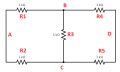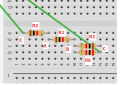#### IonDim

Joined Jun 23, 2023
7
I am trying to create a wheatstone bridge and I have a question about something. Say I have the circuit below. Can this be considered a balanced bridge? So that I can take 2x 2k ohms in parallel and recreate a circuit on the breadboard that will be more simplified?

#### ericgibbs

Joined Jan 29, 2010
18,268
Hi IOn,
Welcome to AAC,
It would be a bridge , if it had say 5Vdc on the left side of the circuit and the 0v on the other.
Is that what you are asking.?

E

#### IonDim

Joined Jun 23, 2023
7
Hi IOn,
Welcome to AAC,
It would be a bridge , if it had say 5Vdc on the left side of the circuit and the 0v on the other.
Is that what you are asking.?

E
Hello eric, and thanks for the quick reply. My circuit is like the image i attach. I am just wondering if that can be considered a wheatstone bridge and if it is balanced, which means I get a simpler circuit that has 4 resistors where 2 and 2 are in series and then they are in parallel.

Ion

#### crutschow

Joined Mar 14, 2008
33,369
I get a simpler circuit that has 4 resistors where 2 and 2 are in series and then they are in parallel.
Post a schematic of your "simpler circuit".

#### Danko

Joined Nov 22, 2017
1,776
So that I can take 2x 2k ohms in parallel and recreate a circuit on the breadboard that will be more simplified?
Oh, yes!
Only do not forget to take out enamel insulation from each 2 kΩ resistor
and connect your power line "+V" exactly in the middle of one 2 kΩ resistor
and connect line "0" exactly in the middle of other 2 kΩ resistor.
That's it.

#### ericgibbs

Joined Jan 29, 2010
18,268
hi Ion,
As this is homework I can only give you guidance, not direct answers, OK.

If the 4 outer 1k resistors are say the exact same resistance value, then Va Vb voltage will be 0V, so the inner 1k is not required.

E

#### IonDim

Joined Jun 23, 2023
7
Alright so I can simplify my circuit like this, since there is no voltage from Va to Vb and maybe replace those 2 parallel 2k ohm resistors with 1 1k ohm one. I just hope I am not missing something or did a mistake regarding the theory.

#### ericgibbs

Joined Jan 29, 2010
18,268
hi Ion,
It is no longer a Wheatstone bridge, just two resistors in parallel.
E

Consider, where would you be able to take off an imbalance voltage signal.???

Hint: consider Va, Vb in my modified circuit.

#### BobTPH

Joined Jun 5, 2013
8,149
I don’t understand the purpose of this question. If there is no connection to the output of the bridge, it call all be replaced by a single resistor, no matter what the values of the resistors are.

#### crutschow

Joined Mar 14, 2008
33,369
Alright so I can simplify my circuit like this, since there is no voltage from Va to Vb and maybe replace those 2 parallel 2k ohm resistors with 1 1k ohm one. I just hope I am not missing something or did a mistake regarding the theory.
Yes, a fundamental misunderstanding.

The output of interest in a Wheatstone bridge is the voltage that appears across the two sets of resistors to show whether the bridge is balanced or not.
It is 0V when balanced, but non-zero otherwise.

You can't have a Wheatstone bridge with less than four elements.

Last edited:

#### WBahn

Joined Mar 31, 2012
29,522
Hello eric, and thanks for the quick reply. My circuit is like the image i attach. I am just wondering if that can be considered a wheatstone bridge and if it is balanced, which means I get a simpler circuit that has 4 resistors where 2 and 2 are in series and then they are in parallel.
I think you are confusing a couple of things and, in turn, creating confusion amongst some of the readers.

It sounds, to me, like your actual task is to find the equivalent resistance, as seen between the left and right nodes, of the collection of resistors given.

What you are asking is whether, like in a balanced Wheatstone bridge circuit, that the bridging resistance can be ignored because there is no voltage across it and, hence, the equivalent resistance can be found by removing it entirely.

The answer to that is yes, you can do it in this special case.

That does not make the circuit a Wheatstone bridge circuit, but merely a circuit that you are utilizing a concept, or a trick, that is commonly exploited in a Wheatstone bridge circuit.

#### WBahn

Joined Mar 31, 2012
29,522
I don’t understand the purpose of this question. If there is no connection to the output of the bridge, it call all be replaced by a single resistor, no matter what the values of the resistors are.
I think the purpose of the question is to find the value of that single resistor. If the student recognizes the symmetry here, they can do that in a matter of seconds in their head. If they don't, then they get to spend quite a bit more time doing something like applying a test source and finding the resulting test current or doing a delta-wye transformation.

#### Alec_t

Joined Sep 17, 2013
14,012
Hint:
Get into the habit of giving each component a label (e.g. R1, R2 etc) so that it is easier for us to understand what your problem is and to discuss the circuit.

#### IonDim

Joined Jun 23, 2023
7
I had an exercise to find the total Resistance of a circuit but the problem is I cannot create it on the breadboard. What I could do instead was switch the 2 1kOhms resistors that are in series(on the right side) to a 2k Ohm and get the result. What is the way to go with it if I have to use all 5 resistors?

#### IonDim

Joined Jun 23, 2023
7
Just for a quick FYI the 2 parallel resistors are a 2k and 1k Ohm while the other 2 are 1k Ohm

#### WBahn

Joined Mar 31, 2012
29,522
I had an exercise to find the total Resistance of a circuit but the problem is I cannot create it on the breadboard. What I could do instead was switch the 2 1kOhms resistors that are in series(on the right side) to a 2k Ohm and get the result. What is the way to go with it if I have to use all 5 resistors?
Total resistance between what two points? That's a critical piece of information. Don't make people guess, we are not mind readers.

If I had to guess, I would assume that you want the resistance between the left edge and the right edge of the circuit. But that's at odds with the claim that the two 1 kΩ resistors on the right side are in series, because they wouldn't be if that were the case. The solution is not to make us guess.

As for how to put five resistors onto the breadboard, what's the problem?

Make your diagrams easy for people to understand and discuss. Put reference designators and node labels on both so that people can match them up.Now you can say things like you replaced the series combination of R4 and R5 with 2 kΩ resistor (which we'll call R6).R6 HAS to be the 2 kΩ resistor, which means that one side has to be Node B and the other side Node C. Flip a coin. R3 has to be the one in parallel with it, as it's the only remaining resistor connected to both Node B and Node C. Work out what the other nodes and resistors are, and you will see that this circuit is NOT the same as your schematic. You would need a jumper connecting the two Node C strips together and your resistance measurement is then between Node C and Node C, which will be zero.

#### IonDim

Joined Jun 23, 2023
7
Total resistance between what two points? That's a critical piece of information. Don't make people guess, we are not mind readers.

If I had to guess, I would assume that you want the resistance between the left edge and the right edge of the circuit. But that's at odds with the claim that the two 1 kΩ resistors on the right side are in series, because they wouldn't be if that were the case. The solution is not to make us guess.

As for how to put five resistors onto the breadboard, what's the problem?

Make your diagrams easy for people to understand and discuss. Put reference designators and node labels on both so that people can match them up.

View attachment 297234

Now you can say things like you replaced the series combination of R4 and R5 with 2 kΩ resistor (which we'll call R6).

View attachment 297237

R6 HAS to be the 2 kΩ resistor, which means that one side has to be Node B and the other side Node C. Flip a coin. R3 has to be the one in parallel with it, as it's the only remaining resistor connected to both Node B and Node C. Work out what the other nodes and resistors are, and you will see that this circuit is NOT the same as your schematic. You would need a jumper connecting the two Node C strips together and your resistance measurement is then between Node C and Node C, which will be zero.
Ok thanks I got it WBahn. And sorry for not labelling my components. But still if I wanted to make it without the R6(2k Ohms), how can I make 1 resistor be in parallel to 2 others that are in series? From theory I know that the resistor in parallel with the other two in series must have one of its legs in contrast with one resistor and the other leg with the other. For example on the top image if I wanted a resistor in parallel with R1 and R2 I would have to place it in a2 and a10 correct? Again thanks in advance

#### ericgibbs

Joined Jan 29, 2010
18,268
Hi Ion,
Is this what you are asking, the Blue lines are the resistors.
Red in/out.
E

#### IonDim

Joined Jun 23, 2023
7
So I take it that my calculations for solving the circuit are wrong as well? I took:

R4 and R5 in series: R4+R5
In parallel with R3: R3//(R4+R5)
and in series with R1 + R2: R1+R2+R3//(R4+R5)

#### ericgibbs

Joined Jan 29, 2010
18,268
Hi Ion,
As your bridge is balanced, the centre R3 can be ignored in your calculations, it is just the two bridge arms in parallel.

But if the bridge is not balanced, it is more complicated to find the equivalent resistance of the bridge.

Work through this video link, it explains in detail.

E